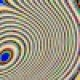Optics

# What are some examples of refraction of light?“The process of bending of light as it passes from air into glass and vice versa is called refraction of light.”Mirage,bent pencil in glass of water,rainbow,sunset are some examples of refraction of light.

## Why does refraction occur?

If we dip one end of a pencil or some other object into water at an angle to the surface, the submerged part looks bent. Its image is displaced because the light coming from the underwater portion of the

object changes direction at it leaves the water. This bending of light as it passed from one transparent medium into another is called refraction.Refraction of light can be explained with the help of figure given below.A ray of light IO travelling from air falls on the surface of a glass block.

At the air glass interface,the ray of light IO changes direction and bends towards the normal and travels along the path OR inside the glass block.The rays IO and OR are called  the incident ray and the refracted ray respectively.The angle ‘i’ made by the incident ray with the normal is called angle of incidence.The angle ‘r’ made by the refractive ray with the normal is called angle of refraction.When refracted ray leaves the glass,it bends away from the normal and travels along the path ME.

## Laws of refraction of light

• The incident ray,the refracted ray,and the normal at the point of incidence all lie in the same plane.
• The ratio of the sine of the angle of incidence ‘i’ to the sine of the angle of refraction ‘r’ is always equal to a constant i.e.,Sin i/Sin r =constant =n

## Snell’s law of refraction

Where the ratio Sin i/Sin r is known as the refractive index of the sound medium with respect to the first medium.So we have:

Sin i/Sin r =n

It is called Snell’s law.

Refraction of light is caused by the difference in speed of light in different media.For example,the speed of light in air is approximately 3.0 × 108 ms-1 ,however when light travels through a medium,such as water or glass,its speed decreases.The speed of light in water is approximately 2.3 × 108 ms-1,while in glass,it is approximately 2.0 ×108 ms-1. To describe the change in the speed of light in a medium,we use the term refractive index.

watch:

## Refractive index

The refractive index ‘n’ of a medium is the ratio of the speed of light ‘c’ in air to the speed ‘v’ of light in the medium.Table of refractive index of some substances is given:

 Substance Refractive index (n) Diamond 2.42 Cubic Zirconia 2.21 Glass (flint) 1.66 Glass (crown) 1.52 Ethyl Alcohol 1.36 Ice 1.31 Water 1.33 Air 1.00

Dispersion of light is due to the variation in refractive index with the colour. Dispersion in drops of water separates the colours of sunlight into rainbow.

## Refraction of light through Prism

Prism is the transparent object (made of optical glass) with at least two polished plane faces inclined towards each other from which light is refracted.In case of triangular prism, the emergent ray is not parallel to the incident ray. It is deviated by the prism from its original path. The incident ray PE makes an angle of incidence ‘i’ at point E and is refracted towards the normal N as EF.  The refracted ray EF makes an angle ‘r’ inside the prism and travels to the other face of the prism. This ray emerges out from the prism at point F making an angle ‘e’. Hence the emerging ray FS is not parallel to the incident ray PE but it deviated by an angle D which is called angle of deviation.

### One Comment

1.Robert J Smith says:

An excellent site. I only wish I had known about it long ago.
Please keep it up and thank you from an old man who still likes to learn.
Bob

Check Also
Close